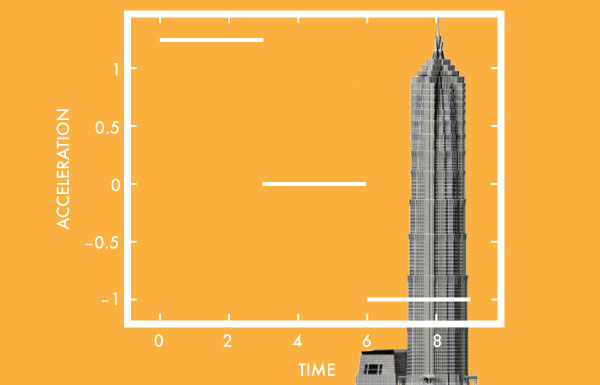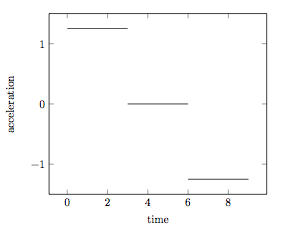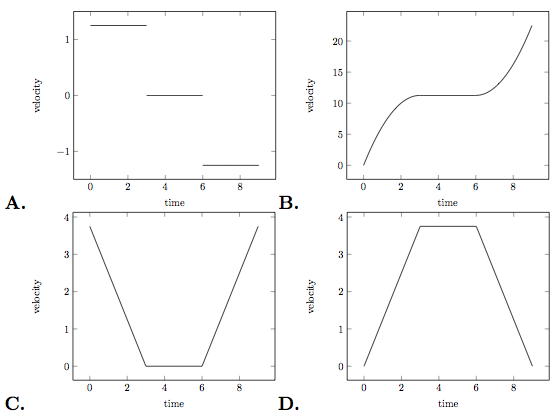Calculus

# Displacement, Velocity, Acceleration (Derivatives): Level 2 Challenges

Which of the following is closest to the magnitude of the average velocity of Earth relative to the sun over the course of one year?The Shanghai Tower has an architectural height of 632 meters, and is equipped with the world's fastest elevator which can travel at $18$ m/s! If we assume that it travels at it's maximum speed, then it would take just under 36 seconds to reach the top. However, this assumption is unrealistic, as the elevator needs time to increase its velocity, i.e. accelerate.

If the acceleration of the elevator is given by the following graph:Which of the following is the best graph to illustrate the velocity of the elevator?A particle is thrown straight up with a velocity of $20 \text{ m/sec}$. Find the time (in seconds) from the start of motion at which the distance traveled is twice the displacement.

Use $g=10~m/s^{2}$.

A jogger is jogging around a circular park of radius 1 kilometer at a speed of 6.28 kilometers per hour. The jogger starts at some point $A$, and jogs around the park to a point $B$, diametrically opposite to $A$. During his jog, how many minutes after starting from $A$, does the ratio between the jogger's distance and the jogger's displacement reach a maximum?

Details:

Take $\pi\approx 3.14$

The ratio to be maximized is $\frac{Distance}{Displacement}$

A train driver is driving along and he suddenly puts on the brakes. After she holds the brakes for $t$ seconds, the train's sliding distance can be measured as $d(t) = 18t - \frac{3}{4} t^2$ (in meters), till it comes to a stop. How far (in meters) did it take for the train take to stop, after the brakes were applied?

×